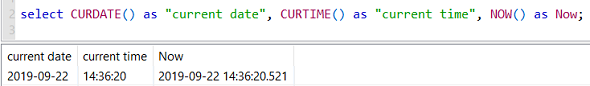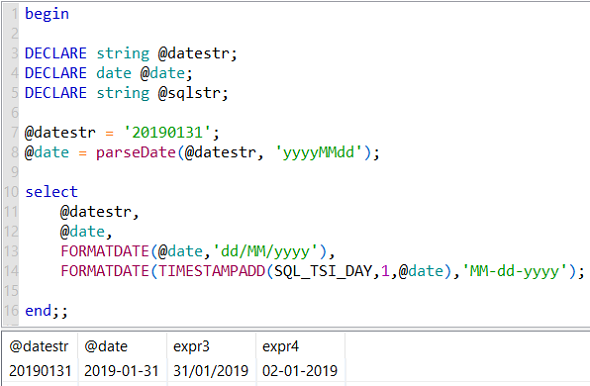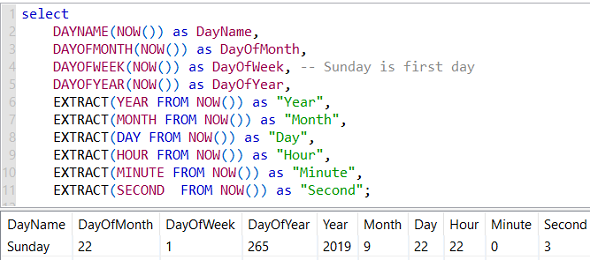Amazon Web Services AWS Tutorials and Guides Development resources, articles, tutorials, code samples and tools and downloads for ASP.Net, SQL Server, R Script, Windows, Windows Phone, AWS, SAP HANA and ABAP, like SAP UI5, Screen Personas, etc.
 Home Articles News IT Jobs Tools Sample Chapters Trainers Blogs Forums Photos Files

# Data Virtuality SQL Date and Time Functions

In this SQL tutorial for Data Virtuality logical datawarehouse developer, I want to share a few SQL codes that might help to convert date strings into date variables or vice versa and add date period to an existing date variable. It is a common task for all SQL programmers of all data platforms to convert a date string into a date variable or display a date variable in different formats like dd-mm-yyyy or mm/dd/yyyy, etc. I will try to show the date time functions for Data Virtuality for SQL programmers that will be useful for them in their daily tasks.

Here is the most frequently used date time functions for SQL programmers.
I think the SQL date and time functions are self explaining.
In order to get current date and time values, according to your requirement you can use one of the CURDATE current date, CURTIME current time or NOW functions.

``` select CURDATE() as "current date", CURTIME() as "current time", NOW() as Now; ```Our second SQL sample assumes that a string variable representing a date value in YYYYMMDD format is an input to our code.
First of all, database developer will convert the string value into an other variable of date data type.
As the second step, the SQL developer will convert the date into string variable with a predefined format "dd/MM/yyyy"
Finally, we will add a date period to our calculated date variable and display it in a different date format.

Here is the SQL code block you can execute on your Data Virtuality instance.
The input date string variable is @datestr
Using ParseDate function, you can convert the string value into a date value. Here is the date format argument is important.
After developer has the date value in @date variable, using FormatDate function the date value can be displayed in different formats like "dd/MM/yyyy" in our example.
Another SQL DateTime function TimestampAdd for Data Virtuality developers enables to add a date period to an input date variable.
In below code, the last line adds 1 day to @date variable value using TimestampAdd() function and then displays the calculated date in a given date format of 'MM-dd-yyyy' by using FormatDate() function.
Please pay attention to SQL_TSI_DAY input argument to TimestampAdd() SQL function which defines the added period as Days.

``` begin DECLARE string @datestr; DECLARE date @date; DECLARE string @sqlstr; @datestr = '20190131'; @date = parseDate(@datestr, 'yyyyMMdd'); select  @datestr,  @date,  FormatDate(@date,'dd/MM/yyyy'),  FormatDate(TimestampAdd(SQL_TSI_DAY,1,@date),'MM-dd-yyyy'); end;; ```

If you execute above SQL script on a Data Virtuality instance, the output will be as follows:SQL developers can use TimestampAdd function to add a certain amount of interval of datetime period.
TimestampAdd function takes three input variables; interval, count, and timestamp value.
TimeStampAdd function can be thought as an equivalent function of DateAdd function on SQL Server.

Possible values for input interval argument can be one of following:
SQL_TSI_FRAC_SECOND: for fractional seconds (billionths of a second),
SQL_TSI_SECOND: for seconds,
SQL_TSI_MINUTE: for minutes,
SQL_TSI_HOUR: for hours,
SQL_TSI_DAY: for days,
SQL_TSI_WEEK: for weeks,
SQL_TSI_MONTH: for months,
SQL_TSI_QUARTER: for quarters,
SQL_TSI_YEAR: for years

## Extract Data from Date Time Variable using SQL Functions

SQL developers can use a number of datetime functions in order to extract valuable information from datetime variable.

For example, it is possible to get the name of current time using DayName() function. Or programmers can use Extract() function by passing two arguments like "Hour" and current datetime value to fetch the current hour value.

Following SQL Select statement demonstrates use of a number of SQL DateTime functions``` select  DAYNAME(NOW()) as DayName,  DAYOFMONTH(NOW()) as DayOfMonth,  DAYOFWEEK(NOW()) as DayOfWeek, -- Sunday is first day  DAYOFYEAR(NOW()) as DayOfYear,  EXTRACT(YEAR FROM NOW()) as "Year",  EXTRACT(MONTH FROM NOW()) as "Month",  EXTRACT(DAY FROM NOW()) as "Day",  EXTRACT(HOUR FROM NOW()) as "Hour",  EXTRACT(MINUTE FROM NOW()) as "Minute",  EXTRACT(SECOND FROM NOW()) as "Second"; ```

Just like DayName() function MonthName() function also exists which returns the name of the current month if NOW() datetime variable is passed as an input argument.

``` select MonthName(NOW()) as "Month Name"; ```

SQL developers working on Data Virtuality Logical Datawarehouse platform can refer to Reference Guide for Date_Time functions.

AWS Tutorials

AWS Tutorials

AWS